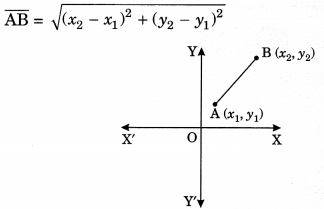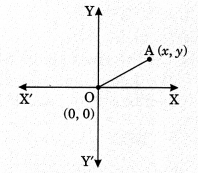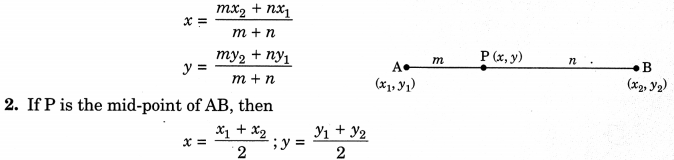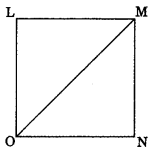On this page, you will find Coordinate Geometry Class 10 Notes Maths Chapter 7 Pdf free download. CBSE NCERT Class 10 Maths Notes Chapter 7 Coordinate Geometry will seemingly help them to revise the important concepts in less time.

## CBSE Class 10 Maths Chapter 7 Notes Coordinate Geometry

### Coordinate Geometry Class 10 Notes Understanding the Lesson

Distance formula

1. The distance between two points A(x1, y1) and B(x2, y2) is2. The distance of point A(x, y) from origin 0(0, 0) is
$$\mathrm{AO}=\sqrt{x^{2}+y^{2}}$$3. Three given points will form:

• Right angled triangle if sum of squares of any two sides is equal to the square of third (largest) side.
• Equilateral triangle if length of all three sides are equal.
• Isosceles triangle if length of any two sides are equal.
• A line or collinear if sum of two sides is equal to third side.

4. Four given points will form:

• Square if length of all four sides are equal and diagonals are equal.
• Rhombus if length of all four sides are equal.
• Rectangle if opposite sides are equal and diagonals are equal.
• Parallelogram if opposite sides are equal.

Section formula
I. If A(x1, y1) and BB(x2, y2)) are two points on a plane and P(x, y) divides AB internally in the ratio m : n, then co-ordinates of P are given byArea of a Triangle

1. Area of ΔABC formed by vertices A(x1, y1), B(x2, y2) and C(x3, y3) is given by
Ar(ΔABC) =$$\frac{1}{2}$$ (- y3) + x2 (y3 – y1)+ x3(y1 – y2)]
[Only positive numerical value to be taken]

2. If Ar(ΔABC) = 0, then A, B and C are collinear points.3. If‘C’ is centroid of a triangle, the median is divided in the ratio 2 : 1 by C and coordinates of C are
$$\left(\frac{x_{1}+x_{2}+x_{3}}{3}, \frac{y_{1}+y_{2}+y_{3}}{3}\right)$$

4. Area of quadrilateral LMNO = ar(ΔLMO) + ar(ΔNMO)